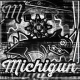# CAST THE ODD ONE OUT (is this among us)

Enigma 1695 states (credit to Enigmatic Code and New Scientist):

“In the following list of five numbers I have consistently replaced digits by letters, with different letters being used for different digits: CAST THE ODD ONE OUT. All but one of those five numbers is a perfect square. What is the numerical value of the odd one out?”

I worked on this for about a total of one hour.

First, let’s consider some common letters. CAST, THE, OUT. They all use the letter T at either the beginning or the end. The second common case, ODD, ONE, OUT. This one’s fairly interesting. They’re all three-digit numbers with the same beginning, O. And the more interesting thing is the number ODD. In the case that it is a perfect square, it’s either 100, 144, 400, or 900.

I looked through every three-digit square number.

Out of all of them, I crossed out any square number that couldn’t fit into the category of ODD, ONE or OUT.

But, since only one number is the odd one out, at least two of ODD, ONE and OUT are squares. For O’s with only one square or O’s with squares that share a digit other than O, the value of O can’t count. So the O values, 4, 6 and 8 are invalid. New table:

For O = 1, ODD can be one of 100, 144 and either ONE or OUT is either 169 or 196, not both. That leads to the fact that two of three of ODD ONE OUT are squares, but never three. That means that one of the three are not square.

The instant first thing I looked for was if OUT was not square. That was because ONE shared the same last digit as THE. Here’s what I did for ten minutes straight.

I listed every four-digit square numbers for CAST.

If ODD was a square, then O is either 1 or 9. That means ONE is either 169, 196 or 961. Looking through every possible value for THE, I found that if E = 9, then THE = 289, 529 or 729. If E = 6, then THE = 256 or 576. If E = 1, then THE = 841. We can rule out 289, 256, 729 and 841, because the T’s at the beginning of the numbers are not possible ones-digit square numbers. That leaves 529 and 576. Either way, T = 5. The only four-digit square numbers ending with 5 that have no repeating digits are 3025 and 9025. That leaves A as 0, S as 2, T as 5.

Tables so far:

But ONE is either 169 or 196, which means C can’t be 9. Therefore, C = 3. Since S = 2, THE can’t be 529. Therefore, it is 576. As ONE is 1_6, it is 196. N = 9. From here, it is obvious.

I can confirm that it is not true for the other cases.

Well, kinda.

This was supposed to be a test post due to one of the comments on a past blog post, but it became a full post.

Who knows?

## 2 thoughts on “CAST THE ODD ONE OUT (is this among us)”

1.Entity_2C says:

this is a test